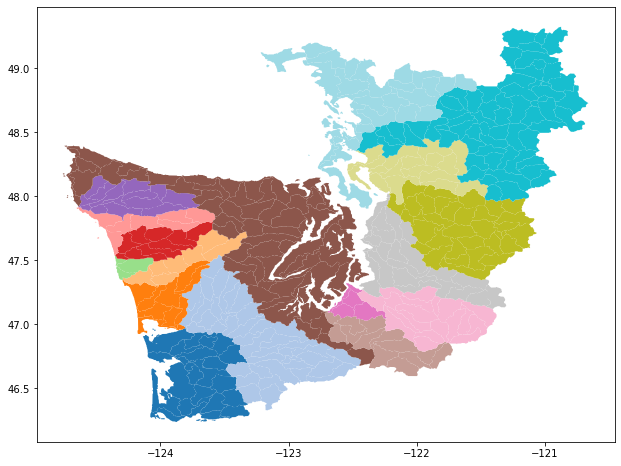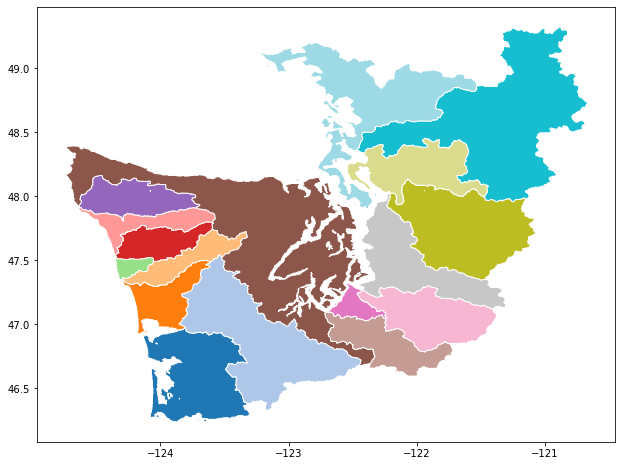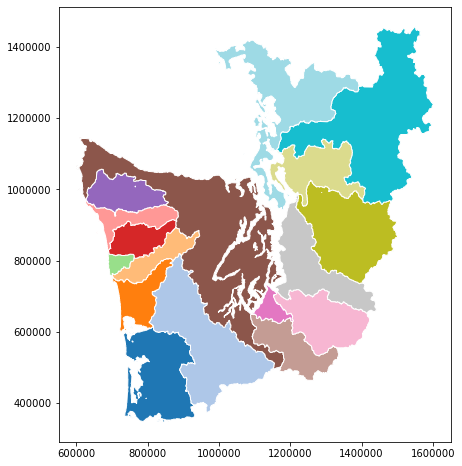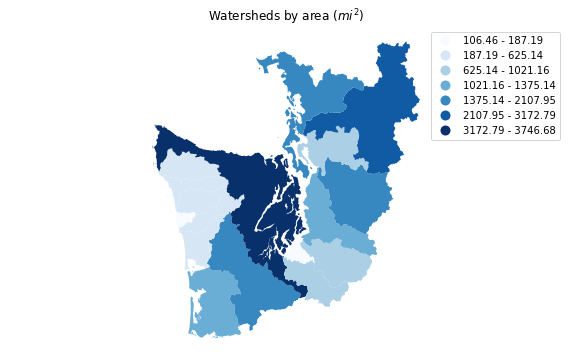## 1. Introduction¶

We covered the basics of GeoPandas in the previous episode and notebook. Here, we'll extend that introduction to illustrate additional aspects of GeoPandas and its interactions with other Python libraries, covering fancier mapping, reprojection, analysis (unitary and binary spatial operators), and raster zonal stats + GeoPandas.

Main sections in this episode / notebook:

• Read HydroBASINS watersheds dataset for Western Washington from a PostGIS / PostgreSQL relational database on the cloud
• Dissolve into larger watersheds, and reproject
• Plot choropleth map based on calculated watershed areas
• Choropleth map as an interactive map with folium
• Spatial join, sjoin, of polygons on points
• rasterstats: "zonal" statistics from polygons on rasters

## 2. Import packages, and set data file path and database connection credentials¶

In :
from pathlib import Path
import json
import psycopg2

import matplotlib as mpl
import matplotlib.pyplot as plt
%matplotlib inline

from shapely.geometry import Point
import pandas as pd
import geopandas as gpd
from geopandas import GeoSeries, GeoDataFrame

In :
mpl.__version__, pd.__version__, gpd.__version__

Out:
('3.1.1', '0.25.1', '0.5.1')
In :
# On pangeo, uncomment this line to install this package not present in the pangeo conda environment
# !conda install -y -c conda-forge mapclassify

In :
data_pth = Path("../data")

In :
with open(data_pth / "db.json") as f:


## 3. Read HydroBASINS North America dataset, extracting Western Washington¶

Read HydroBASINS "all-levels" (lev00) hierarchical watersheds dataset for North America and the Caribbean (hybas_na_lev00_v1c), from Amazon Cloud PostgreSQL/PostGIS database. Watersheds in the dataset are at the finest (highest resolution) "Pfastetter" hierarchical level, level 12. HydroBASINS dataset technical documentation is here.

read_postgis is called as before, except now we'll apply a SQL filter (server side -- let the server do extra work for you) to the PostGIS dataset to select only the Pfastetter level-4 watershed with code 7831: WHERE pfaf_4 = 7831. This is most of Western Washington. Watershed polygons will still be read at their original level 12 resolution.

For a more in-depth look at interacting with spatial relational databases, see the eScience Institute tutorial [Introduction to SQL and Geospatial Data Processing](https://uwescience.github.io/SQL-geospatial-tutorial/).
In :
conn = psycopg2.connect(**db_conn_dict)


Note that if the PostGIS geometry column (geom_col argument) is not called geometry, we have to know its name in advance. Bummer.

In :
hydrobas_ww = gpd.read_postgis(
"SELECT * FROM hybas_na_lev00_v1c WHERE pfaf_4 = 7831", conn,
geom_col='polygongeom',
coerce_float=False)

In :
conn.close()

In :
hydrobas_ww.crs

Out:
{'init': 'epsg:4326'}
In :
len(hydrobas_ww)

Out:
413

413 polygon features returned. Let's examine the attributes available, using the first feature as an example.

In :
hydrobas_ww.iloc

Out:
gid                                                        19945
hybas_id                                             7.00001e+09
next_down                                                      0
next_sink                                            7.00001e+09
main_bas                                             7.00001e+09
dist_sink                                                      0
dist_main                                                      0
sub_area                                                   135.4
up_area                                                    135.4
endo                                                           0
coast                                                          0
order                                                          1
sort                                                       19945
pfaf_1                                                         7
pfaf_2                                                        78
pfaf_3                                                       783
pfaf_4                                                      7831
pfaf_5                                                     78310
pfaf_6                                                    783101
pfaf_7                                                   7831010
pfaf_8                                                  78310101
pfaf_9                                                 783101010
pfaf_10                                              7.83101e+09
pfaf_11                                              7.83101e+10
pfaf_12                                              7.83101e+11
polygongeom    (POLYGON ((-123.35 46.36666666666669, -123.349...
Name: 0, dtype: object

Plot a categorical map with coloring based on the aggregating column pfaf_7. Watershed boundaries are at the high-resolution Pfastetter level 12.
Note: pick a color map (cmap) appropriate for your data. Get to know the matplotlib color maps.

In :
hydrobas_ww.plot(column='pfaf_7', cmap='tab20', categorical=True, figsize=(14, 8));### Rename the GeoDataFrame geometry column from polygongeom to geometry to avoid issues with other packages¶

Unfortunately, folium choropleth and rasterstats (demonstrated below) require the geometry column to be named "geometry" (as of 2018-9). So, we'll rename it here first.

In :
hydrobas_ww = hydrobas_ww.rename(columns={'polygongeom': 'geometry'})
hydrobas_ww._geometry_column_name = 'geometry'


## 4. Dissolve into larger watersheds, and reproject¶

### Dissolve source polygons into larger watersheds based on attribute values¶

Apply GeoDataFrame dissolve aggregation method (implemented from lower-level shapely operators) on level-7 Pfastetter codes (pfaf_7) shown in the plot above. Aggregate attributes, retaining only pfaf_7 and pfaf_6 (plus geometry, of course).

In :
cols = ['pfaf_6', 'pfaf_7', 'geometry']
hydrobas_ww_p7 = hydrobas_ww[cols].dissolve(by='pfaf_7', aggfunc='first', as_index=False)
len(hydrobas_ww_p7)

Out:
17

This operation resulted in only 17 polygons, from the original 413. Let's examine some of the features.

In :
hydrobas_ww_p7.head()

Out:
pfaf_7 geometry pfaf_6
0 7831010 (POLYGON ((-123.4666666666666 46.2666666666666... 783101
1 7831020 POLYGON ((-123.1791666666666 46.33333333333336... 783102
2 7831031 (POLYGON ((-123.9597222222222 46.9666666666667... 783103
3 7831032 POLYGON ((-123.8583333333333 47.39583333333336... 783103
4 7831033 POLYGON ((-124.3 47.34583333333336, -124.30221... 783103

Plot the results. Looks like the previous plot, except the polygon boundaries are now the pfaf_7 watersheds.

In :
hydrobas_ww_p7.plot(column='pfaf_7', cmap='tab20', categorical=True, edgecolor='white',
figsize=(14, 8));Beware of invalid geometries!
**Beware that *dissolve* may fail if there are "invalid" geometries.** The code below is based on a GeoDataFrame examined in the previous, intro notebook. The 6 geometries/points reported are invalid (and are reported by the *is_valid()* method). This dissolve statement does work, though. python seas_grp = seas[['oceans', 'geometry']] seas_oceans_diss = seas_grp[seas_grp.geometry.is_valid].dissolve(by='oceans') Ring Self-intersection at or near point 10.407218181818182 54.821390909090908 Self-intersection at or near point -79.365827272727287 76.296645454545455 Ring Self-intersection at or near point 10.979445510225332 54.380555030408686 Ring Self-intersection at or near point 133.61550925464189 -4.3005540903175188 Ring Self-intersection at or near point 121.91067196634913 -5.0593090510592447 Ring Self-intersection at or near point 115.29553592754269 -7.0082630551828515 

### Reproject (transform) to WA State Plane South, epsg:2927¶

Partly so we can calculate polygon areas in planar units, not geodetic degrees. But also because that's the projection used by most state and local governments in Washington.

Projections can be explored at various web resources.For example these links rely on different systems to provide information about epsg:2927: - http://epsg.io/2927 - http://spatialreference.org/ref/epsg/2927/ - [Report from http://www.epsg-registry.org](http://www.epsg-registry.org/report.htm?type=selection&entity=urn:ogc:def:crs:EPSG::2927&reportDetail=short&style=urn:uuid:report-style:default-with-code&style_name=OGP%20Default%20With%20Code&title=EPSG:2927) We can extract the epsg code from the GeoDataFrame crs property (from the string returned by crs['init']), then pass it to pyepsg to programmatically obtain information about a projection: python import pyepsg pyepsg.get(hydrobas_ww_p7.crs['init'].split(':')) 

Let's use pyepsg, which issues queries to http://epsg.io web services, to examine the properties of a projection.

In :
import pyepsg
pyepsg.get('2927')

Out:
<ProjectedCRS: 2927, NAD83(HARN) / Washington South (ftUS)>

Apply the crs transformation (reprojection) using to_crs method.

In :
hydrobas_ww_p7_wasp = hydrobas_ww_p7.to_crs(epsg=2927)


Plot the reprojected map. Note that, being in a planar project (not geodetic), the shape looks different compared to the previous map. More "normal". And the axes are now in feet relative to some origin.

In :
hydrobas_ww_p7_wasp.plot(column='pfaf_7', cmap='tab20', categorical=True, edgecolor='white',
figsize=(14, 8));## 5. Plot choropleth map based on calculated watershed areas¶

As the projection is in feet, auto-calculated polygon areas will be in feet2. So let's convert to miles2 first (why not!). We'll add a new column to the GeoDataFrame.

In :
hydrobas_ww_p7_wasp['area_mi2'] = hydrobas_ww_p7_wasp.geometry.area / 27878400

Out:
pfaf_7 geometry pfaf_6 area_mi2
0 7831010 (POLYGON ((890315.2572612339 354459.8899780852... 783101 1375.137396
1 7831020 POLYGON ((963803.8027083555 376154.996568859, ... 783102 2107.945774
2 7831031 (POLYGON ((776917.1877152117 614568.3833321583... 783103 528.472846
Pandas "groupby" aggregation
You could calculate the area of a pfaf_6 watershed via simple Pandas DataFrame *groupby* aggregation (sum).

### Plot the choloropleth, using area_mi2.¶

Choropleth classifiers from PySAL mapclassify
The "fisher_jenks" value segmentation scheme (using 7 segments, k=7) used is one of the available choropleth classifiers from the powerful [PySAL Library (Python Spatial Analysis Library)](http://pysal.org/), specifically from its [mapclassify](https://pysal.org/mapclassify/) package. GeoPandas can use these classifiers if mapclassify is installed, as it is here. To get the list of classifiers, use: python import mapclassify print(mapclassify.CLASSIFIERS) 
In :
f, ax = plt.subplots(1, figsize=(10, 6))
ax.set_title('Watersheds by area ($mi^2$)')
hydrobas_ww_p7_wasp.plot(column='area_mi2', scheme='fisher_jenks', k=7,
cmap=plt.cm.Blues, legend=True, ax=ax)
ax.set_axis_off()
plt.axis('equal');Time to Explore

## 6. Choropleth map as an interactive map with folium¶

Folium is very cool, specially for use in Jupyter notebooks; or to export into stand-alone HTML.

In :
import folium

In :
folium.__version__

Out:
'0.10.0'

m.choropleth internally splits the geometry from the other attributes in hydrobas_ww_p7_wasp, and rejoins them based on the key pfaf_7. key_on uses an attribute reference derived from GeoJSON representations; this is awkward, and hopefully will be simplified in future folium implementations.

In :
hydrobas_ww_p7_wasp.head()

Out:
pfaf_7 geometry pfaf_6 area_mi2
0 7831010 (POLYGON ((890315.2572612339 354459.8899780852... 783101 1375.137396
1 7831020 POLYGON ((963803.8027083555 376154.996568859, ... 783102 2107.945774
2 7831031 (POLYGON ((776917.1877152117 614568.3833321583... 783103 528.472846
3 7831032 POLYGON ((808869.5755557647 769864.5311527678,... 783103 441.528065
4 7831033 POLYGON ((698711.1808079168 756609.8674803785,... 783103 106.456891
In :
m = folium.Map(location=[47.8, -122.5], zoom_start=7, tiles="cartodbpositron")

folium.Choropleth(
geo_data=hydrobas_ww_p7_wasp,
data=hydrobas_ww_p7_wasp,
columns=['pfaf_7', 'area_mi2'],
key_on='feature.properties.pfaf_7',
legend_name='Area (sq mi)',
fill_color='YlGn',
fill_opacity=0.4,
highlight=True

<folium.features.Choropleth at 0x7f722a0d17b8>
m